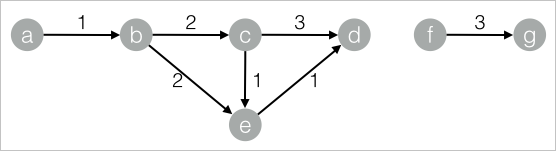The single-source shortest path (SSSP) refers to the shortest path between a vertex and all other vertices. The shortest path is calculated by using the Dijkstra algorithm. This topic describes the Single-source Shortest Path component provided by Machine Learning Studio.

You can configure the component by using one of the following methods:

## Machine Learning Platform for AI console

Tab Parameter Description
Fields Settings Source Vertex Column The start vertex column in the edge table.
Target Vertex Column The end vertex column in the edge table.
Edge Weight Column The edge weight column in the edge table.
Parameters Settings Start Vertex ID The start vertex that is used to calculate the shortest path. This parameter is required.
Tuning Workers The number of vertices for parallel job execution. The parallelism level and framework communication costs increase with the value of this parameter.
Memory Size Per Worker (MB) The maximum size of memory that a single job can use. By default, the system allocates 4,096 MB for each job. If the used memory size exceeds the value of this parameter, the OutOfMemory exception is reported.

## PAI command

``````PAI -name SSSP
-project algo_public
-DinputEdgeTableName=SSSP_func_test_edge
-DfromVertexCol=flow_out_id
-DtoVertexCol=flow_in_id
-DoutputTableName=SSSP_func_test_result
-DhasEdgeWeight=true
-DedgeWeightCol=edge_weight
-DstartVertex=a;``````
Parameter Required Description Default value
inputEdgeTableName Yes The name of the input edge table. No default value
inputEdgeTablePartitions No The partitions in the input edge table. Full table
fromVertexCol Yes The start vertex column in the input edge table. No default value
toVertexCol Yes The end vertex column in the input edge table. No default value
outputTableName Yes The name of the output table. No default value
outputTablePartitions No The partitions in the output table. No default value
lifecycle No The lifecycle of the output table. No default value
workerNum No The number of vertices for parallel job execution. The parallelism level and framework communication costs increase with the value of this parameter. Not configured
workerMem No The maximum size of memory that a single job can use. By default, the system allocates 4,096 MB for each job. If the used memory size exceeds the value of this parameter, the OutOfMemory exception is reported. 4096
splitSize No The data split size. 64
startVertex Yes The ID of the start vertex. No default value
hasEdgeWeight No Specifies whether the edges in the input edge table have weights. false
edgeWeightCol No The edge weight column in the input edge table. No default value

## Examples

1. Generate training data.
``````drop table if exists SSSP_func_test_edge;
create table SSSP_func_test_edge as
select
flow_out_id,flow_in_id,edge_weight
from
(
select "a" as flow_out_id,"b" as flow_in_id,1.0 as edge_weight from dual
union all
select "b" as flow_out_id,"c" as flow_in_id,2.0 as edge_weight from dual
union all
select "c" as flow_out_id,"d" as flow_in_id,1.0 as edge_weight from dual
union all
select "b" as flow_out_id,"e" as flow_in_id,2.0 as edge_weight from dual
union all
select "e" as flow_out_id,"d" as flow_in_id,1.0 as edge_weight from dual
union all
select "c" as flow_out_id,"e" as flow_in_id,1.0 as edge_weight from dual
union all
select "f" as flow_out_id,"g" as flow_in_id,3.0 as edge_weight from dual
union all
select "a" as flow_out_id,"d" as flow_in_id,4.0 as edge_weight from dual
) tmp;``````
The following figure shows the structure of the SSSP graph.2. View training results.
``````+------------+------------+------------+--------------+
| start_node | dest_node  | distance   | distance_cnt |
+------------+------------+------------+--------------+
| a          | b          | 1.0        | 1            |
| a          | c          | 3.0        | 1            |
| a          | d          | 4.0        | 3            |
| a          | a          | 0.0        | 0            |
| a          | e          | 3.0        | 1            |
+------------+------------+------------+--------------+``````